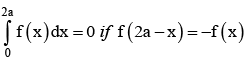# Test: Definite Integral (Competition Level) - 1

## 30 Questions MCQ Test Mathematics (Maths) Class 12 | Test: Definite Integral (Competition Level) - 1

Description
This mock test of Test: Definite Integral (Competition Level) - 1 for JEE helps you for every JEE entrance exam. This contains 30 Multiple Choice Questions for JEE Test: Definite Integral (Competition Level) - 1 (mcq) to study with solutions a complete question bank. The solved questions answers in this Test: Definite Integral (Competition Level) - 1 quiz give you a good mix of easy questions and tough questions. JEE students definitely take this Test: Definite Integral (Competition Level) - 1 exercise for a better result in the exam. You can find other Test: Definite Integral (Competition Level) - 1 extra questions, long questions & short questions for JEE on EduRev as well by searching above.
QUESTION: 1

###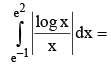Solution:

Given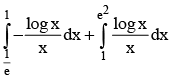QUESTION: 2

### if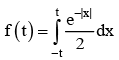then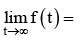Solution:

Even  function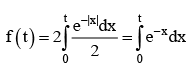QUESTION: 3

###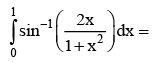Solution: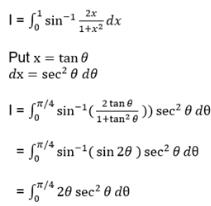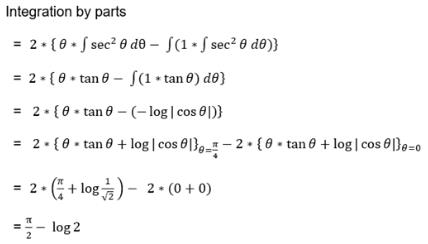QUESTION: 4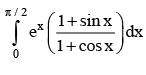Solution:

use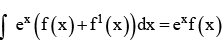QUESTION: 5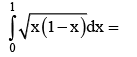Solution: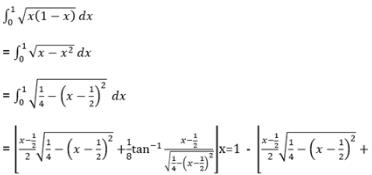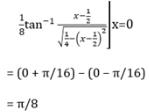QUESTION: 6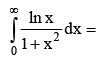Solution:
QUESTION: 7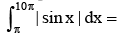Solution: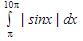Period of |sinx| is π
Therefore, the integration becomes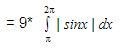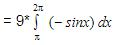= (-9) *(-1)*( [cosx]x=2π – [cosx]x=π)
= 9 * (1 – (-1))
= 18

QUESTION: 8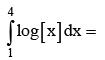Solution:

Given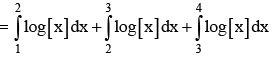QUESTION: 9

if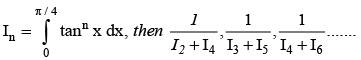​from

Solution: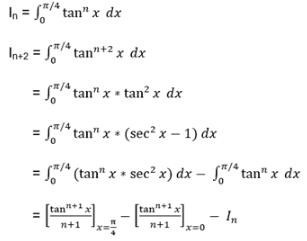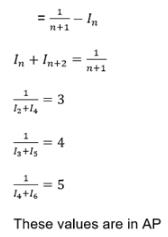QUESTION: 10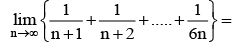Solution: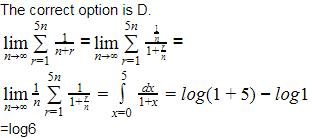QUESTION: 11

If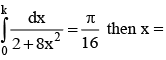Solution:

use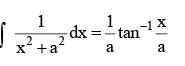QUESTION: 12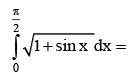Solution: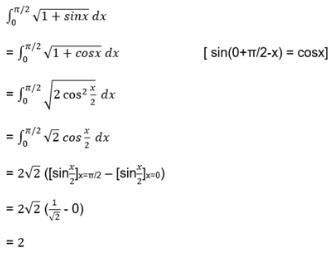QUESTION: 13

If 0 < a < c, 0 < b < c then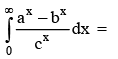Solution: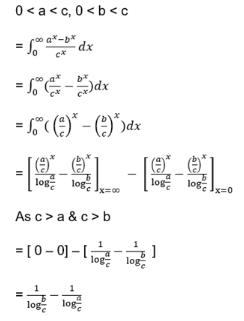QUESTION: 14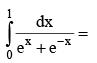Solution: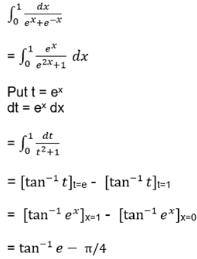QUESTION: 15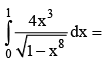Solution:

Put x4 =t

QUESTION: 16

If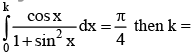Solution:

Sin x = t

QUESTION: 17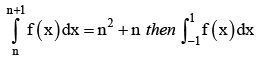Solution:

Split into two integrals

QUESTION: 18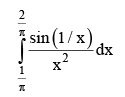Solution:

Put 1/x= t

QUESTION: 19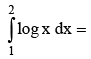Solution: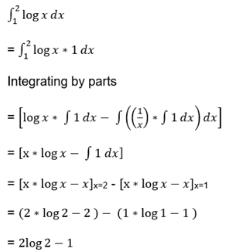QUESTION: 20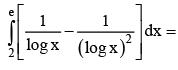Solution:

Log x = t

QUESTION: 21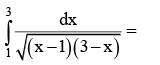Solution: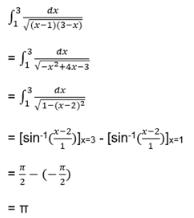QUESTION: 22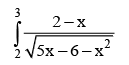Solution: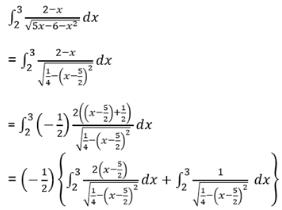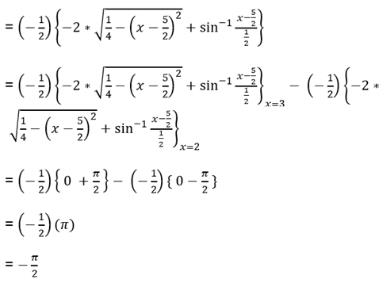QUESTION: 23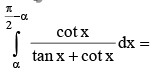Solution: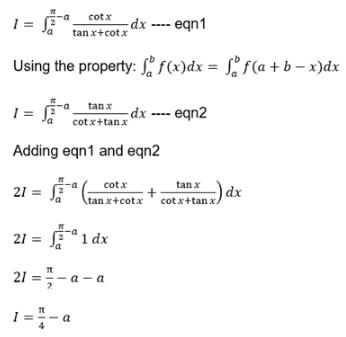QUESTION: 24

If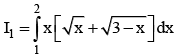and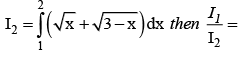Solution: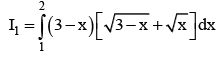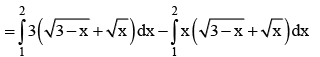QUESTION: 25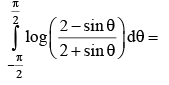Solution:

Given function is odd

QUESTION: 26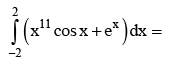Solution:

x11 cos x is an odd function.

QUESTION: 27

The function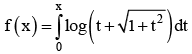is

Solution:

Solution we know that if f(t) is an odd function the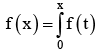dt is given integral since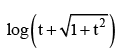is odd, the given integral is even

QUESTION: 28

Value of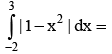Solution:

Given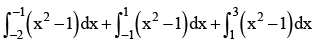QUESTION: 29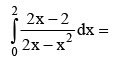Solution:

use formula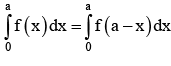QUESTION: 30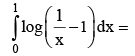Solution:

Using